# RD Sharma Solutions for Class 8 Maths Chapter 13 Profit, Loss, Discount and Value Added Tax (VAT) Exercise 13.3

To meet the article expenditures, the government imposes different types of taxes. Value Added Tax (VAT) is one of these. The VAT is calculated on the selling price, if a discount is given. If there is no discount then VAT is calculated on the marked (list) price of the article. In Exercise 13.3 of Chapter 13, we shall discuss problems based on value-added tax (VAT). RD Sharma Solutions which is formulated by our tutors at BYJU’S is the best study material one can use to secure high marks in their examination. Students wishing to build their confidence level can download the pdf given in the links below, and by practising the solutions on a regular basis can achieve good scores in their exams.

## Download the Pdf of RD Sharma Solutions for Class 8 Maths Exercise 13.3 Chapter 13 Profit, Loss, Discount and Value Added Tax (VAT)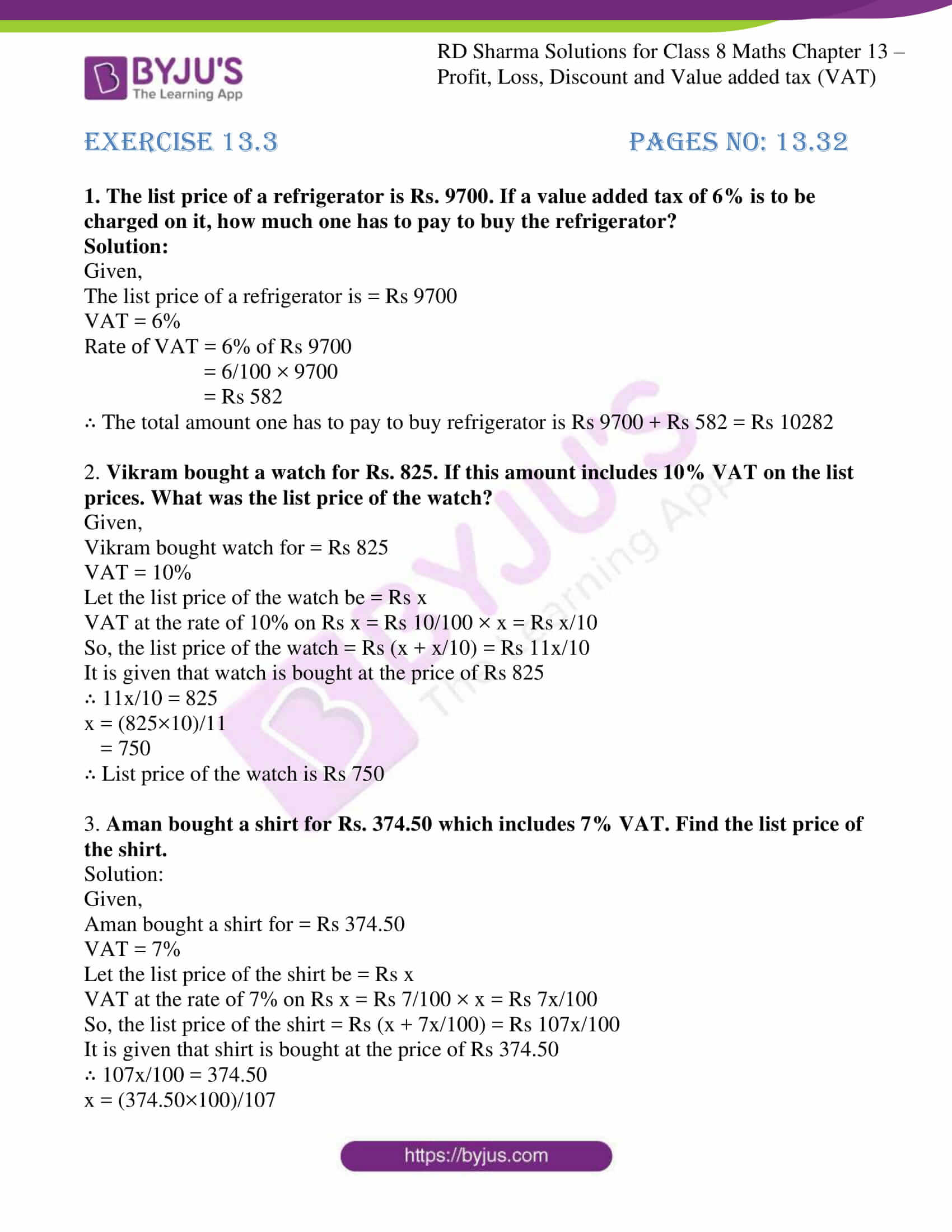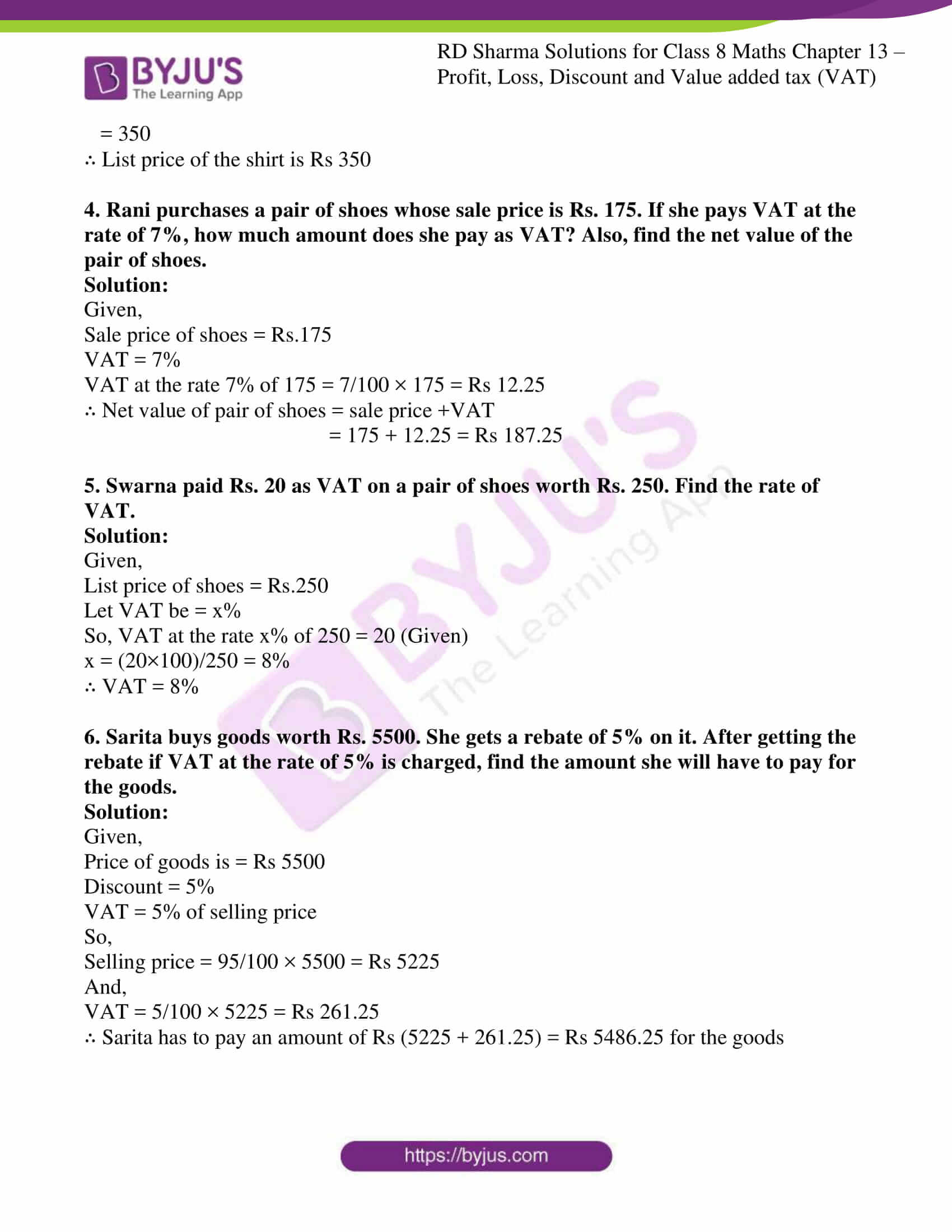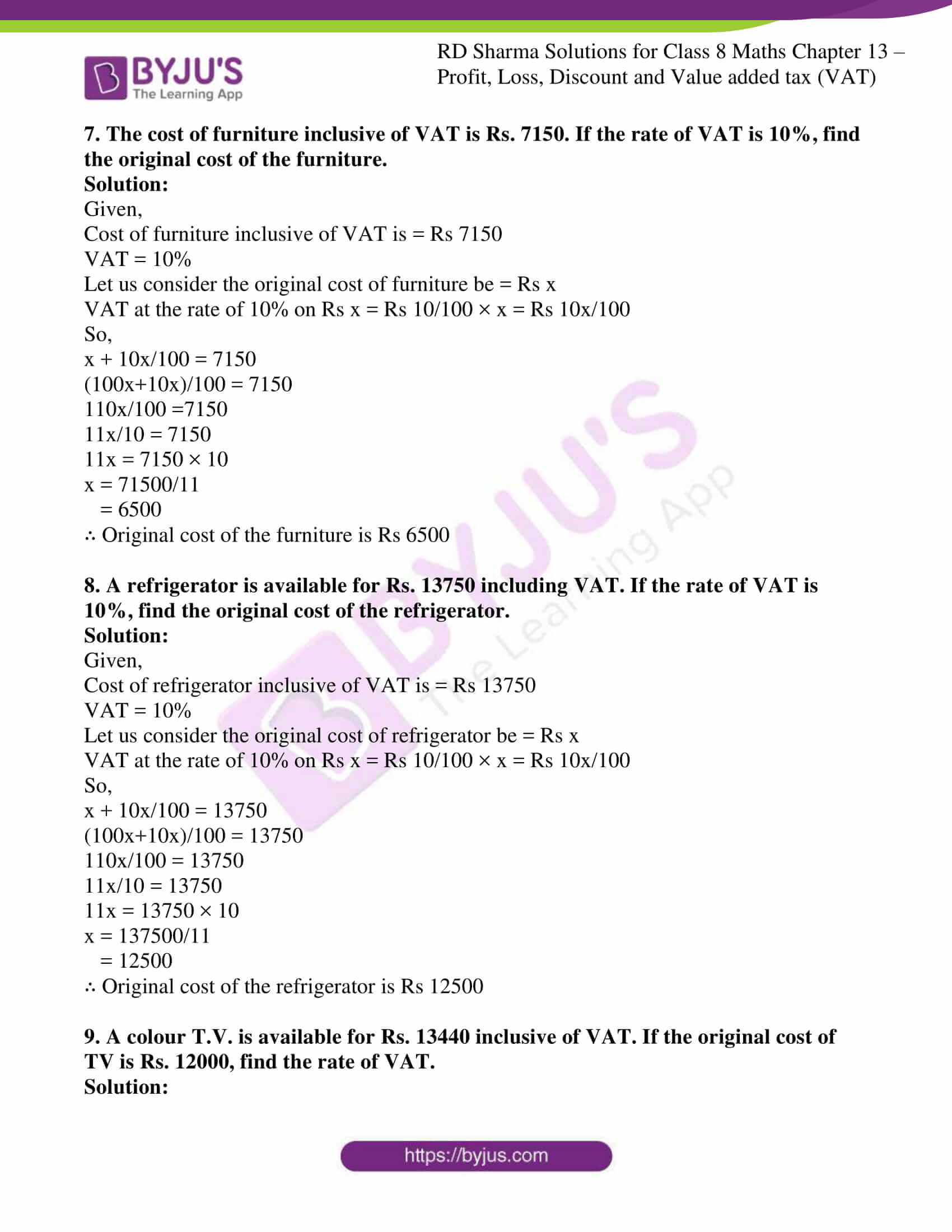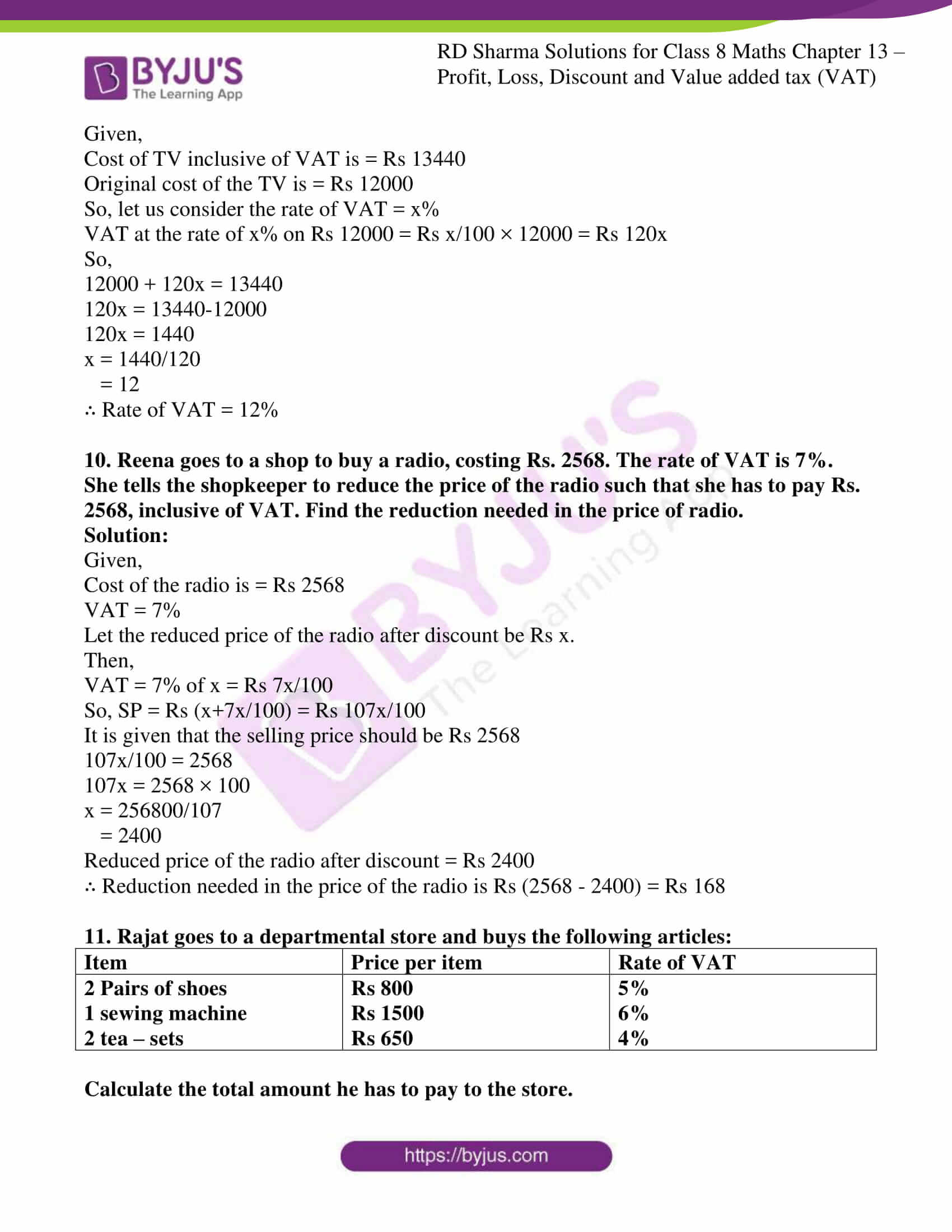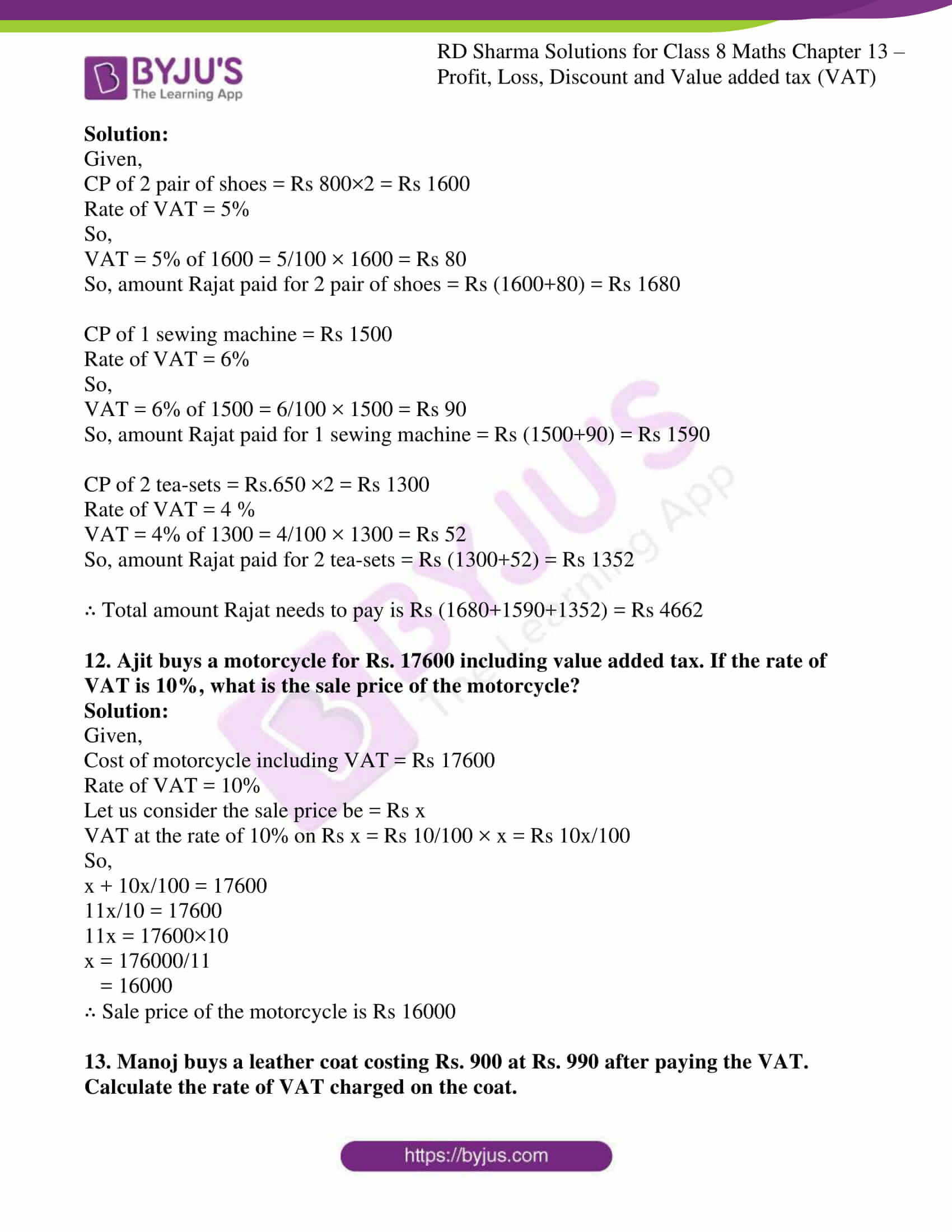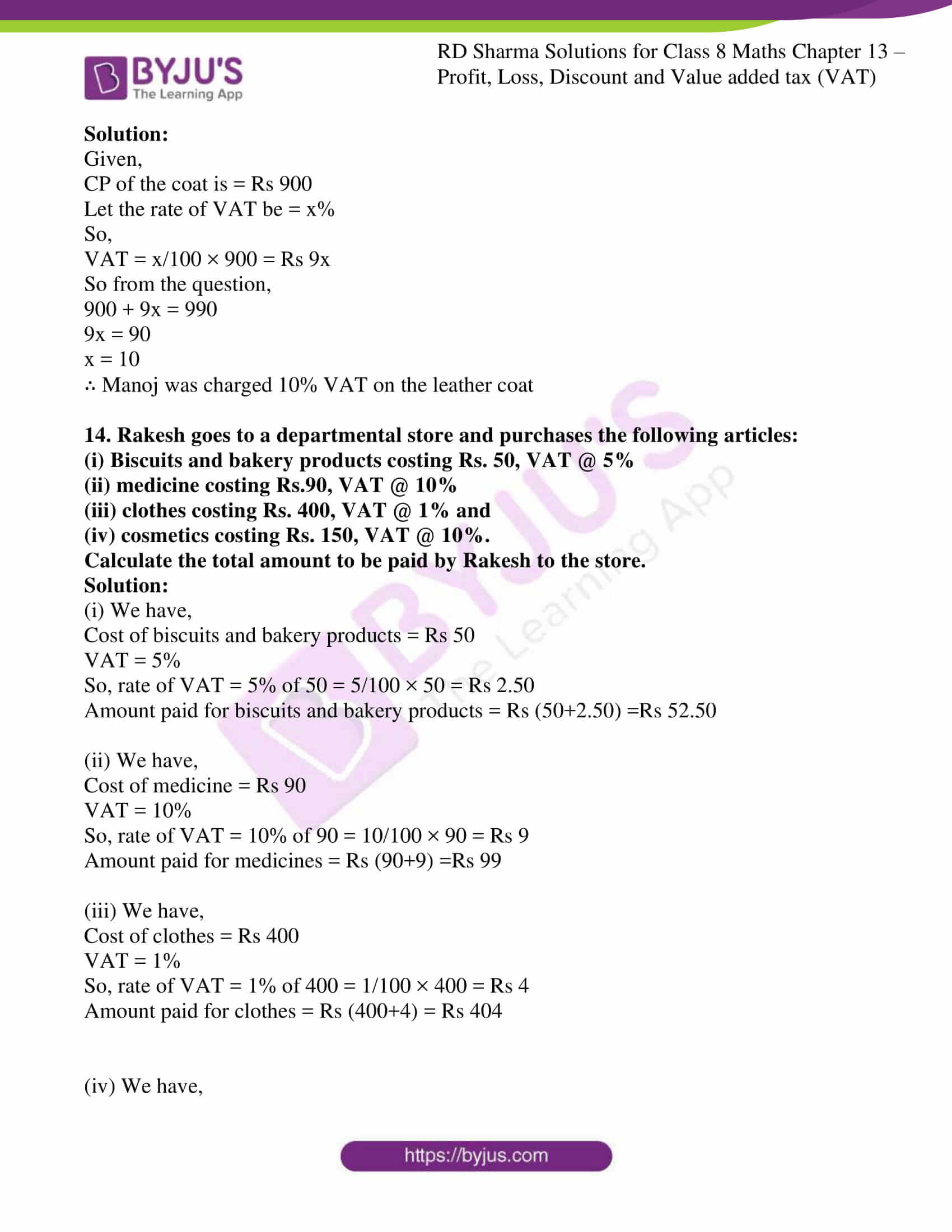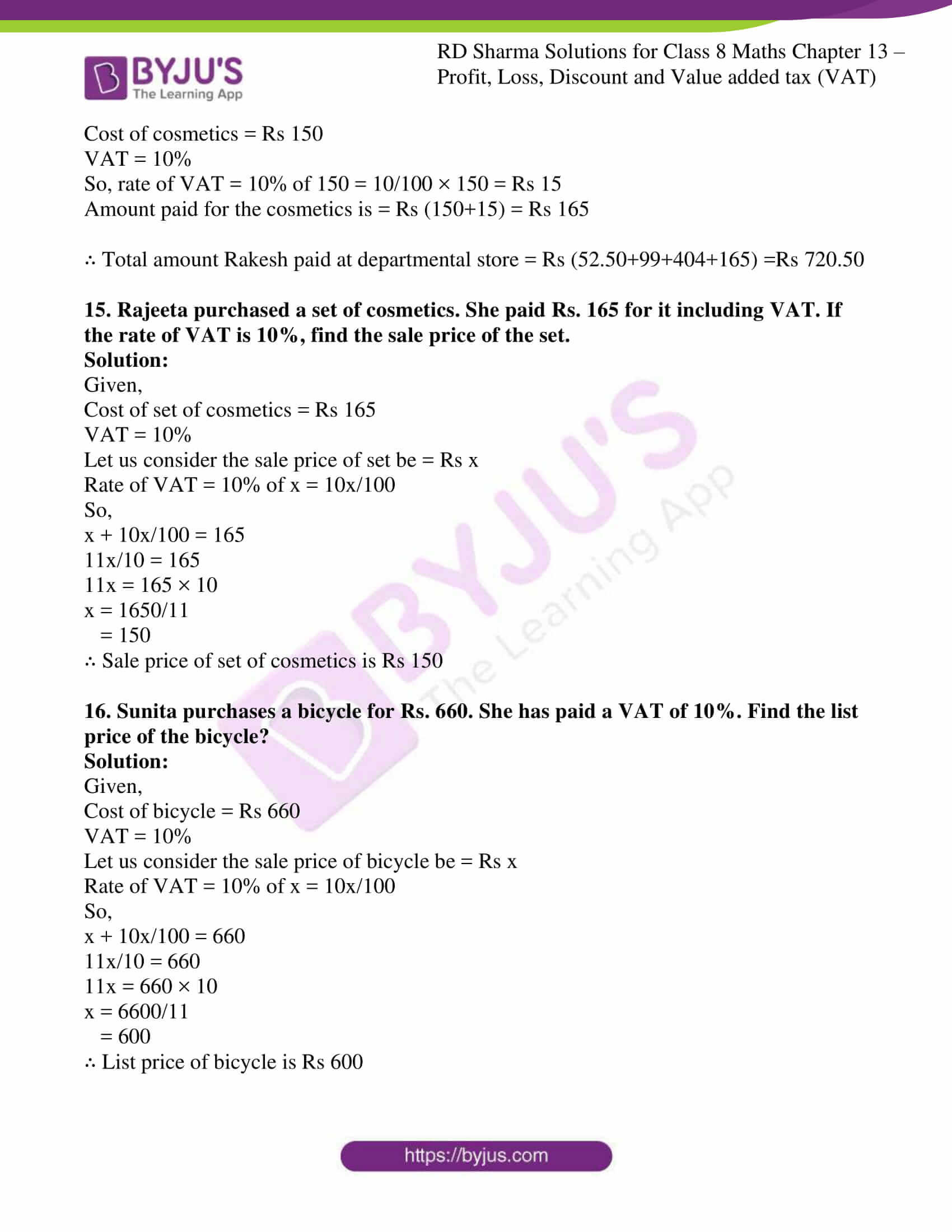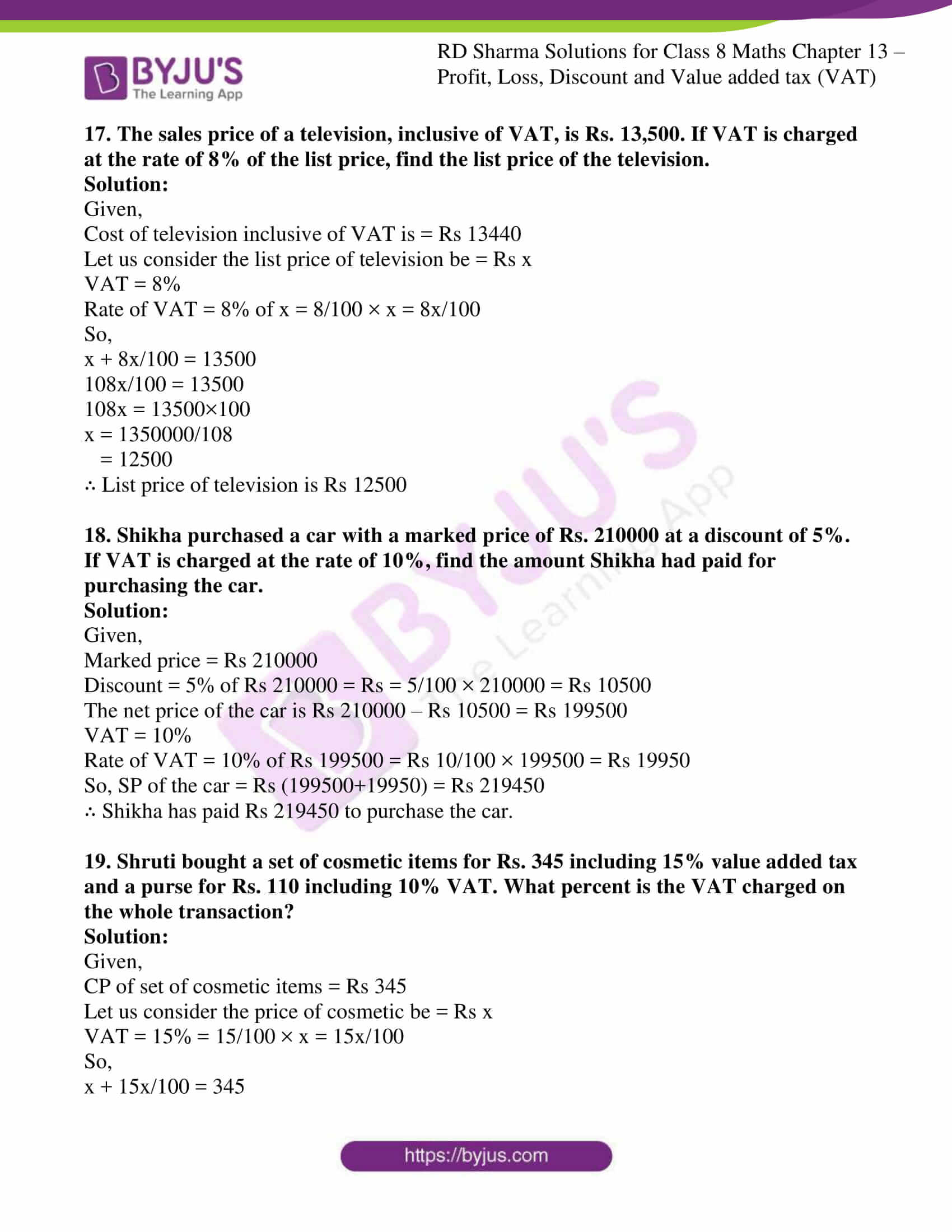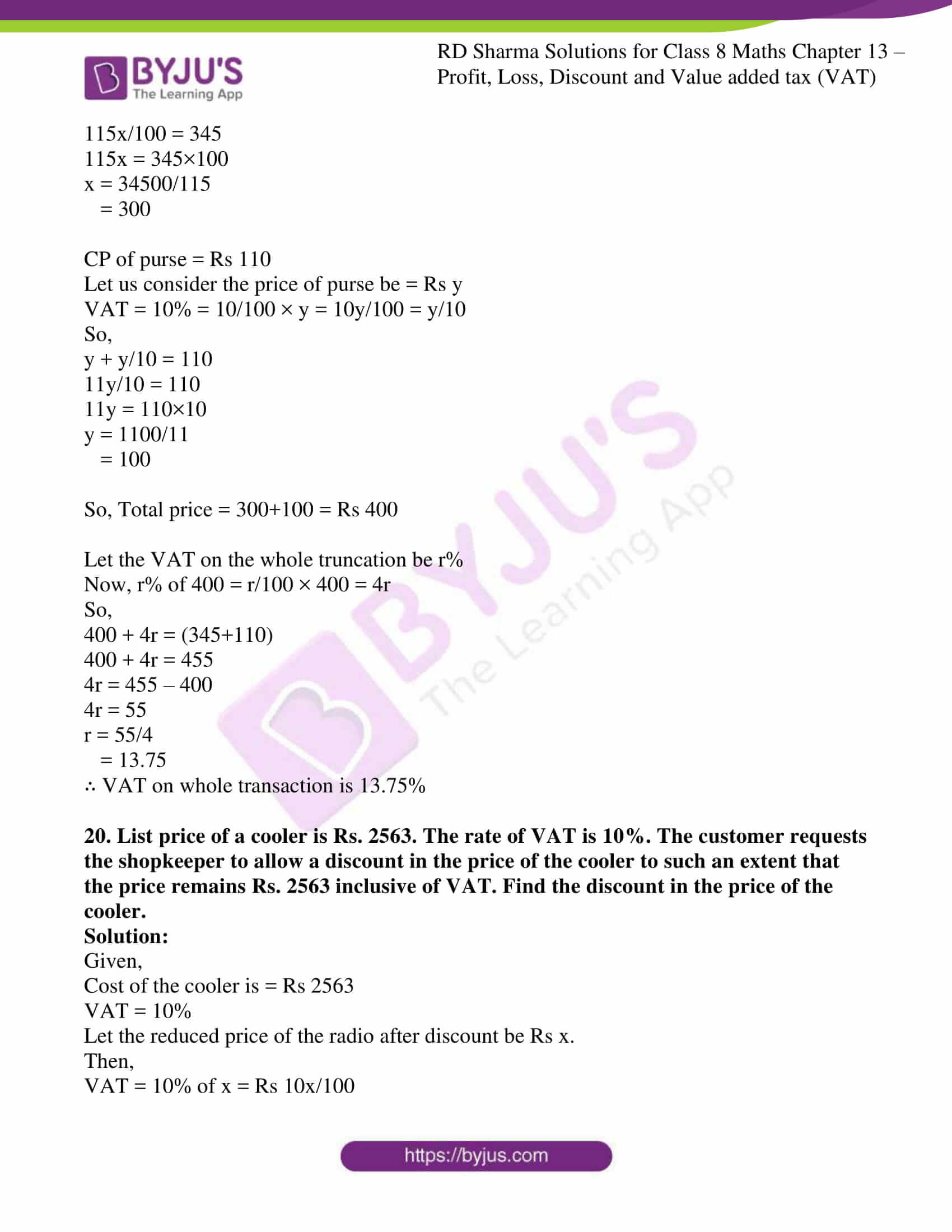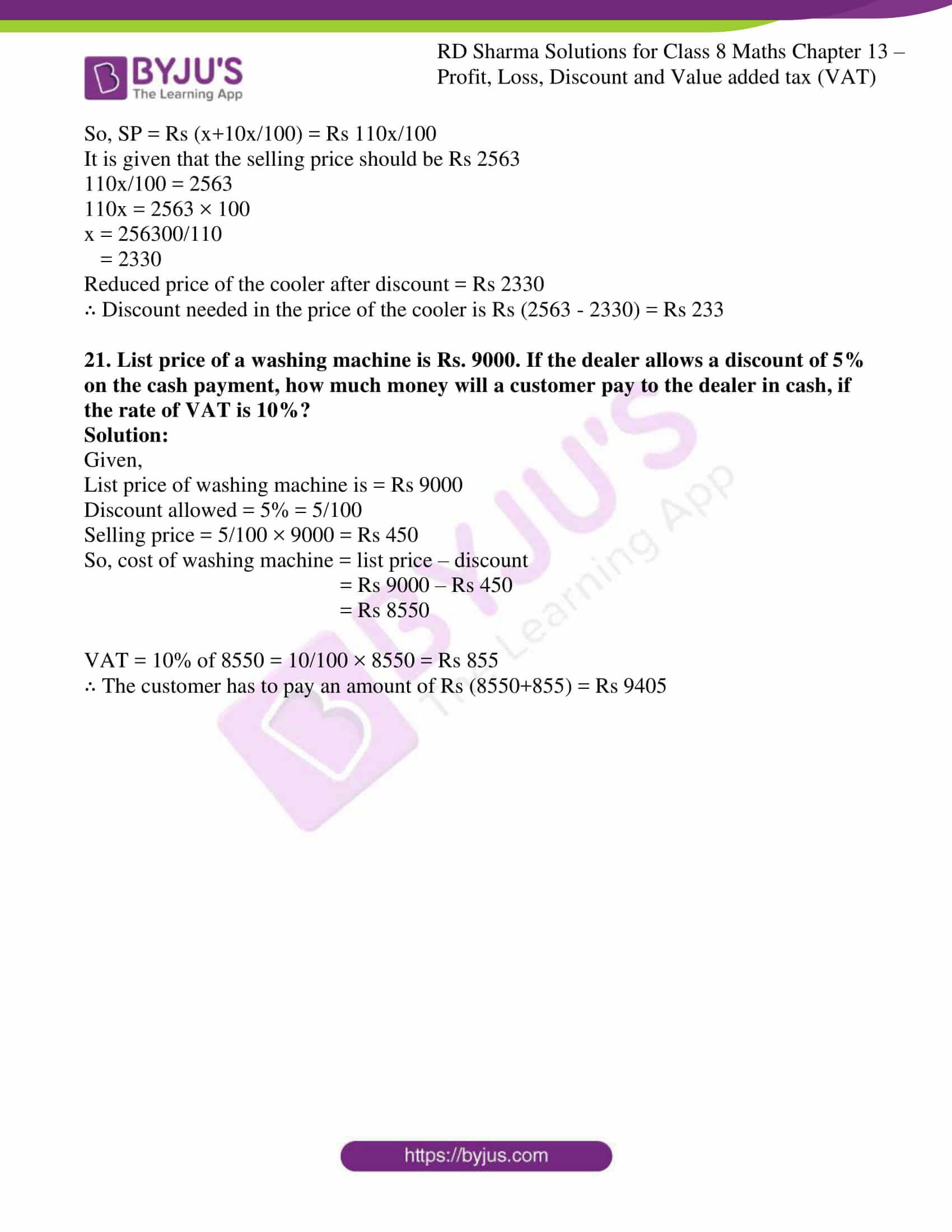### Access answers to Maths RD Sharma Solutions For Class 8 Exercise 13.3 Chapter 13 Profit, Loss, Discount and Value Added Tax (VAT)

1. The list price of a refrigerator is Rs. 9700. If a value added tax of 6% is to be charged on it, how much one has to pay to buy the refrigerator?

Solution:

Given,

The list price of a refrigerator is = Rs 9700

VAT = 6%

Rate of VAT = 6% of Rs 9700

= 6/100 × 9700

= Rs 582

∴ The total amount one has to pay to buy refrigerator is Rs 9700 + Rs 582 = Rs 10282

2. Vikram bought a watch for Rs. 825. If this amount includes 10% VAT on the list prices. What was the list price of the watch?

Given,

Vikram bought watch for = Rs 825

VAT = 10%

Let the list price of the watch be = Rs x

VAT at the rate of 10% on Rs x = Rs 10/100 × x = Rs x/10

So, the list price of the watch = Rs (x + x/10) = Rs 11x/10

It is given that watch is bought at the price of Rs 825

∴ 11x/10 = 825

x = (825×10)/11

= 750

∴ List price of the watch is Rs 750

3. Aman bought a shirt for Rs. 374.50 which includes 7% VAT. Find the list price of the shirt.

Solution:

Given,

Aman bought a shirt for = Rs 374.50

VAT = 7%

Let the list price of the shirt be = Rs x

VAT at the rate of 7% on Rs x = Rs 7/100 × x = Rs 7x/100

So, the list price of the shirt = Rs (x + 7x/100) = Rs 107x/100

It is given that shirt is bought at the price of Rs 374.50

∴ 107x/100 = 374.50

x = (374.50×100)/107

= 350

∴ List price of the shirt is Rs 350

4. Rani purchases a pair of shoes whose sale price is Rs. 175. If she pays VAT at the rate of 7%, how much amount does she pay as VAT? Also, find the net value of the pair of shoes.

Solution:

Given,

Sale price of shoes = Rs.175

VAT = 7%

VAT at the rate 7% of 175 = 7/100 × 175 = Rs 12.25

∴ Net value of pair of shoes = sale price +VAT

= 175 + 12.25 = Rs 187.25

5. Swarna paid Rs. 20 as VAT on a pair of shoes worth Rs. 250. Find the rate of VAT.

Solution:

Given,

List price of shoes = Rs.250

Let VAT be = x%

So, VAT at the rate x% of 250 = 20 (Given)

x = (20×100)/250 = 8%

∴ VAT = 8%

6. Sarita buys goods worth Rs. 5500. She gets a rebate of 5% on it. After getting the rebate if VAT at the rate of 5% is charged, find the amount she will have to pay for the goods.

Solution:

Given,

Price of goods is = Rs 5500

Discount = 5%

VAT = 5% of selling price

So,

Selling price = 95/100 × 5500 = Rs 5225

And,

VAT = 5/100 × 5225 = Rs 261.25

∴ Sarita has to pay an amount of Rs (5225 + 261.25) = Rs 5486.25 for the goods

7. The cost of furniture inclusive of VAT is Rs. 7150. If the rate of VAT is 10%, find the original cost of the furniture.

Solution:

Given,

Cost of furniture inclusive of VAT is = Rs 7150

VAT = 10%

Let us consider the original cost of furniture be = Rs x

VAT at the rate of 10% on Rs x = Rs 10/100 × x = Rs 10x/100

So,

x + 10x/100 = 7150

(100x+10x)/100 = 7150

110x/100 =7150

11x/10 = 7150

11x = 7150 × 10

x = 71500/11

= 6500

∴ Original cost of the furniture is Rs 6500

8. A refrigerator is available for Rs. 13750 including VAT. If the rate of VAT is 10%, find the original cost of the refrigerator.

Solution:

Given,

Cost of refrigerator inclusive of VAT is = Rs 13750

VAT = 10%

Let us consider the original cost of refrigerator be = Rs x

VAT at the rate of 10% on Rs x = Rs 10/100 × x = Rs 10x/100

So,

x + 10x/100 = 13750

(100x+10x)/100 = 13750

110x/100 = 13750

11x/10 = 13750

11x = 13750 × 10

x = 137500/11

= 12500

∴ Original cost of the refrigerator is Rs 12500

9. A colour T.V. is available for Rs. 13440 inclusive of VAT. If the original cost of TV is Rs. 12000, find the rate of VAT.

Solution:

Given,

Cost of TV inclusive of VAT is = Rs 13440

Original cost of the TV is = Rs 12000

So, let us consider the rate of VAT = x%

VAT at the rate of x% on Rs 12000 = Rs x/100 × 12000 = Rs 120x

So,

12000 + 120x = 13440

120x = 13440-12000

120x = 1440

x = 1440/120

= 12

∴ Rate of VAT = 12%

10. Reena goes to a shop to buy a radio, costing Rs. 2568. The rate of VAT is 7%. She tells the shopkeeper to reduce the price of the radio such that she has to pay Rs. 2568, inclusive of VAT. Find the reduction needed in the price of radio.

Solution:

Given,

Cost of the radio is = Rs 2568

VAT = 7%

Let the reduced price of the radio after discount be Rs x.

Then,

VAT = 7% of x = Rs 7x/100

So, SP = Rs (x+7x/100) = Rs 107x/100

It is given that the selling price should be Rs 2568

107x/100 = 2568

107x = 2568 × 100

x = 256800/107

= 2400

Reduced price of the radio after discount = Rs 2400

∴ Reduction needed in the price of the radio is Rs (2568 – 2400) = Rs 168

11. Rajat goes to a departmental store and buys the following articles:

 Item Price per item Rate of VAT 2 Pairs of shoes 1 sewing machine 2 tea – sets Rs 800 Rs 1500 Rs 650 5% 6% 4%

Calculate the total amount he has to pay to the store.

Solution:

Given,

CP of 2 pair of shoes = Rs 800×2 = Rs 1600

Rate of VAT = 5%

So,

VAT = 5% of 1600 = 5/100 × 1600 = Rs 80

So, amount Rajat paid for 2 pair of shoes = Rs (1600+80) = Rs 1680

CP of 1 sewing machine = Rs 1500

Rate of VAT = 6%

So,

VAT = 6% of 1500 = 6/100 × 1500 = Rs 90

So, amount Rajat paid for 1 sewing machine = Rs (1500+90) = Rs 1590

CP of 2 tea-sets = Rs.650 ×2 = Rs 1300

Rate of VAT = 4 %

VAT = 4% of 1300 = 4/100 × 1300 = Rs 52

So, amount Rajat paid for 2 tea-sets = Rs (1300+52) = Rs 1352

∴ Total amount Rajat needs to pay is Rs (1680+1590+1352) = Rs 4662

12. Ajit buys a motorcycle for Rs. 17600 including value added tax. If the rate of VAT is 10%, what is the sale price of the motorcycle?

Solution:

Given,

Cost of motorcycle including VAT = Rs 17600

Rate of VAT = 10%

Let us consider the sale price be = Rs x

VAT at the rate of 10% on Rs x = Rs 10/100 × x = Rs 10x/100

So,

x + 10x/100 = 17600

11x/10 = 17600

11x = 17600×10

x = 176000/11

= 16000

∴ Sale price of the motorcycle is Rs 16000

13. Manoj buys a leather coat costing Rs. 900 at Rs. 990 after paying the VAT. Calculate the rate of VAT charged on the coat.

Solution:

Given,

CP of the coat is = Rs 900

Let the rate of VAT be = x%

So,

VAT = x/100 × 900 = Rs 9x

So from the question,

900 + 9x = 990

9x = 90

x = 10

∴ Manoj was charged 10% VAT on the leather coat

14. Rakesh goes to a departmental store and purchases the following articles:

(i) Biscuits and bakery products costing Rs. 50, VAT @ 5%
(ii) medicine costing Rs.90, VAT @ 10%
(iii) clothes costing Rs. 400, VAT @ 1% and
(iv) cosmetics costing Rs. 150, VAT @ 10%.
Calculate the total amount to be paid by Rakesh to the store.

Solution:

(i) We have,

Cost of biscuits and bakery products = Rs 50

VAT = 5%

So, rate of VAT = 5% of 50 = 5/100 × 50 = Rs 2.50

Amount paid for biscuits and bakery products = Rs (50+2.50) =Rs 52.50

(ii) We have,

Cost of medicine = Rs 90

VAT = 10%

So, rate of VAT = 10% of 90 = 10/100 × 90 = Rs 9

Amount paid for medicines = Rs (90+9) =Rs 99

(iii) We have,

Cost of clothes = Rs 400

VAT = 1%

So, rate of VAT = 1% of 400 = 1/100 × 400 = Rs 4

Amount paid for clothes = Rs (400+4) = Rs 404

(iv) We have,

Cost of cosmetics = Rs 150

VAT = 10%

So, rate of VAT = 10% of 150 = 10/100 × 150 = Rs 15

Amount paid for the cosmetics is = Rs (150+15) = Rs 165

∴ Total amount Rakesh paid at departmental store = Rs (52.50+99+404+165) =Rs 720.50

15. Rajeeta purchased a set of cosmetics. She paid Rs. 165 for it including VAT. If the rate of VAT is 10%, find the sale price of the set.

Solution:

Given,

Cost of set of cosmetics = Rs 165

VAT = 10%

Let us consider the sale price of set be = Rs x

Rate of VAT = 10% of x = 10x/100

So,

x + 10x/100 = 165

11x/10 = 165

11x = 165 × 10

x = 1650/11

= 150

∴ Sale price of set of cosmetics is Rs 150

16. Sunita purchases a bicycle for Rs. 660. She has paid a VAT of 10%. Find the list price of the bicycle?

Solution:

Given,

Cost of bicycle = Rs 660

VAT = 10%

Let us consider the sale price of bicycle be = Rs x

Rate of VAT = 10% of x = 10x/100

So,

x + 10x/100 = 660

11x/10 = 660

11x = 660 × 10

x = 6600/11

= 600

∴ List price of bicycle is Rs 600

17. The sales price of a television, inclusive of VAT, is Rs. 13,500. If VAT is charged at the rate of 8% of the list price, find the list price of the television.

Solution:

Given,

Cost of television inclusive of VAT is = Rs 13440

Let us consider the list price of television be = Rs x

VAT = 8%

Rate of VAT = 8% of x = 8/100 × x = 8x/100

So,

x + 8x/100 = 13500

108x/100 = 13500

108x = 13500×100

x = 1350000/108

= 12500

∴ List price of television is Rs 12500

18. Shikha purchased a car with a marked price of Rs. 210000 at a discount of 5%. If VAT is charged at the rate of 10%, find the amount Shikha had paid for purchasing the car.

Solution:

Given,

Marked price = Rs 210000

Discount = 5% of Rs 210000 = Rs = 5/100 × 210000 = Rs 10500

The net price of the car is Rs 210000 – Rs 10500 = Rs 199500

VAT = 10%

Rate of VAT = 10% of Rs 199500 = Rs 10/100 × 199500 = Rs 19950

So, SP of the car = Rs (199500+19950) = Rs 219450

∴ Shikha has paid Rs 219450 to purchase the car.

19. Shruti bought a set of cosmetic items for Rs. 345 including 15% value added tax and a purse for Rs. 110 including 10% VAT. What percent is the VAT charged on the whole transaction?

Solution:

Given,

CP of set of cosmetic items = Rs 345

Let us consider the price of cosmetic be = Rs x

VAT = 15% = 15/100 × x = 15x/100

So,

x + 15x/100 = 345

115x/100 = 345

115x = 345×100

x = 34500/115

= 300

CP of purse = Rs 110

Let us consider the price of purse be = Rs y

VAT = 10% = 10/100 × y = 10y/100 = y/10

So,

y + y/10 = 110

11y/10 = 110

11y = 110×10

y = 1100/11

= 100

So, Total price = 300+100 = Rs 400

Let the VAT on the whole truncation be r%

Now, r% of 400 = r/100 × 400 = 4r

So,

400 + 4r = (345+110)

400 + 4r = 455

4r = 455 – 400

4r = 55

r = 55/4

= 13.75

∴ VAT on whole transaction is 13.75%

20. List price of a cooler is Rs. 2563. The rate of VAT is 10%. The customer requests the shopkeeper to allow a discount in the price of the cooler to such an extent that the price remains Rs. 2563 inclusive of VAT. Find the discount in the price of the cooler.

Solution:

Given,

Cost of the cooler is = Rs 2563

VAT = 10%

Let the reduced price of the radio after discount be Rs x.

Then,

VAT = 10% of x = Rs 10x/100

So, SP = Rs (x+10x/100) = Rs 110x/100

It is given that the selling price should be Rs 2563

110x/100 = 2563

110x = 2563 × 100

x = 256300/110

= 2330

Reduced price of the cooler after discount = Rs 2330

∴ Discount needed in the price of the cooler is Rs (2563 – 2330) = Rs 233

21. List price of a washing machine is Rs. 9000. If the dealer allows a discount of 5% on the cash payment, how much money will a customer pay to the dealer in cash, if the rate of VAT is 10%?

Solution:

Given,

List price of washing machine is = Rs 9000

Discount allowed = 5% = 5/100

Selling price = 5/100 × 9000 = Rs 450

So, cost of washing machine = list price – discount

= Rs 9000 – Rs 450

= Rs 8550

VAT = 10% of 8550 = 10/100 × 8550 = Rs 855

∴ The customer has to pay an amount of Rs (8550+855) = Rs 9405